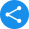# RS Aggarwal Class 9 Solutions for MathsView Notes

The RS Aggarwal Solutions for Class 9 Maths have been provided here for the benefit of the CBSE Class 9 students. All the exercise questions of Maths Class 9 Chapters are solved and it will be a great help for the students in their exam preparation and revision. Vedantu.com is a No.1 online tutoring company in India. These solutions have chapter wise details that are provided to you for Free in PDF format. You will also get a PDF download option for all the RS Aggarwal Solutions that will help you in your exam preparation for academic year 2020-21. Download RS Aggarwal Textbook Solutions for Class 9 Maths from Vedantu, which are curated by master teachers. Also, revise and solve the important questions for the Class 9 Maths (RS Aggarwal) exam using the updated CBSE textbook solutions provided by us.

Vedantu is a platform that provides free NCERT Solutions and other study materials for students. Maths Students who are looking for better solutions .Science Students who are looking for NCERT Solutions for Class 9 Science will also find the Solutions curated by our Master Teachers really Helpful.

### RS Aggarwal Solutions Class 9 - Chapter-wise Solutions for Maths

Q 1: How many chapters are there in class 9 RS Aggarwal solutions book?

Ans: There are a total of 19 chapters in RD Sharma Class 10 maths solutions book. The first part of the consists of chapter 1: Number systems, chapter 2: Polynomials, chapter 3: factorization of polynomials, chapter 4: Linear equations in two variables, chapter 5: coordinate geometry, chapter 6: Introduction to Euclid’s geometry, chapter 7: Lines and angles and chapter 8: triangles.

The later part of the chapter consists of chapter 9: congruence of triangles and inequalities in a triangle, chapter 10: quadrilaterals, chapter 11: areas of a parallelogram and triangles, chapter 12: circles, chapter 13: geometrical construction, chapter 14: area of triangles and quadrilaterals, chapter 15: volume and surface area of solids, chapter 16: presentation of data in tabular form, chapter 17: bar graph, histogram and frequency polygon, chapter 18: mean, median and mode of ungrouped data and chapter 19: probability.

Q 2: How many exercises are there in Class 9 Maths of Chapter 3?

Ans: There are a total of 7 exercises at the end of the chapter. Below is the list of questions in each exercise.

• Exercise 3.1 consists of 34 questions. This exercise is completely based on factor and factorization.

• Exercise 3.2 consists of 40 questions. This exercise focuses on Methods of factorisation.

• Exercise 3.3 consists of 66 questions. Questions in this exercise are based on Factorisation by taking out the common factor.

• Exercise 3.4 consists of 7 questions. This exercise is based on the factorisation by grouping, Factorising the difference of two squares and Factorisation of quadratic trinomials.

• Exercise 3.5 consists of 10 questions. Questions in this exercise are focused on Square of a trinomial.

• Exercise 3.6 consists of 38 questions. This exercise comprises questions from Cube of a binomial concept.

• Exercise 3.7 consists of 25 questions. Questions in this exercise are based on the Factorisation of sum or difference of cubes.

Q 3: Define the following Angle, Interior of an angle, Obtuse angle, Reflex angle, Complementary angles and Supplementary angles.

Ans:

• Angle – When two rays originate from the same endpoint, then an angle is formed.

• Interior of an Angle – The intramural of ∠BAC is the set of all points in its plane which rest on the same side of the line AB as C and also on the same side of AC as B.

• Obtuse Angle – An angle that measures more than 900 but less than 1800 is known as an obtuse angle.

• Reflex Angle – An angle that measures more than 1800 but less than 3600 is known as a reflex angle.

• Complementary Angles – Two angles are denoted to be reciprocal if the sum of their measure is 900.

• Supplementary Angles – Two angles are said to be supplementary if the sum of their measures is 1800.

Q 4: How will Vedantu’s RS Aggarwal solutions guide me?

Ans: Our RS Aggarwal Solutions are the perfect study material for the students of Class 9. The solutions provided by us will help them gain a better understanding of various characteristics related to Maths. All our Solutions are written in a very simple language to make better use of it.

RS Aggarwal Solutions for Class 9 maths will help the students to ace the exam. The substantial practice will make sure that the students write the exams with more confidence. This will aid the students in evaluating their comprehension completely. The solutions provided by us will help you in gaining knowledge and create a strong foundation with the concepts.

Share this with your friendsShare